## NCERT Solutions for Class 9 Science Chapter 11 Work, Power and Energy

These Solutions are part of NCERT Solutions for Class 9 Science. Here we have given NCERT Solutions for Class 9 Science Chapter 11 Work, Power and Energy. LearnInsta.com provides you the Free PDF download of NCERT Solutions for Class 9 Science (Physics) Chapter 11 – Work and Energy solved by Expert Teachers as per NCERT (CBSE) Book guidelines. All Chapter 11 – Work and Energy Exercise Questions with Solutions to help you to revise complete Syllabus and Score More marks.

More Resources

NCERT TEXT BOOK QUESTIONS

IN TEXT QUESTIONS

Question 1.
A force of 7 N acts on an object. The displacement is say 8 m in the direction of the force. Let us take it that the force acts on the object through the displacement. What is the work done in this case ?
(CBSE 2011)
Here, Force, F = 7 N
Displacement, S = 8 m
Work done = F x S = 7 x 8 = 56 J.

Question 2.
When do we say that work is done ? (CBSE 2012)
Work is done, when force acts on an object and the object is displaced from its initial position.

Question 3.
Write an expression for the work done when a force is acting on an object in the direction of its displacement.
W = FS

Question 4.
Define 1 J of work. (CBSE 2012)

Or

Define SI unit of work. (CBSE Sample Paper 2010 ; CBSE 2011, 2012)
Work is said to be 1 J if 1N force displaces an object through 1 metre in its own direction.

Question 5.
A pair of bullocks exerts a force of 140 N on a plough. The field being ploughed is 15 m long. How much work is done in ploughing the length of the field ? (CBSE 2010)
Here, Force, F = 140 N
Displacement, S = 15 m
Work done = F x S = 140 x 15 = 2100 J.

Question 6.
What is the kinetic energy of an object ? (CBSE 2011, 2012, 2014, 2015)
The energy possessed by an object by virtue of its motion is known as the kinetic energy of the object.

Question 7.
Write an expression for the kinetic energy of an object.
K.E. of an object = 1/2 mv2 , where m is the mass of the object and v is the speed of the object.

Question 8.
The kinetic energy of an object of mass m moving with a velocity of 5 m s-1 is 25 J. What will be its kinetic energy when its velocity is doubled ? What will be its kinetic energy when its velocity is increased
(CBSE 2010, 2012)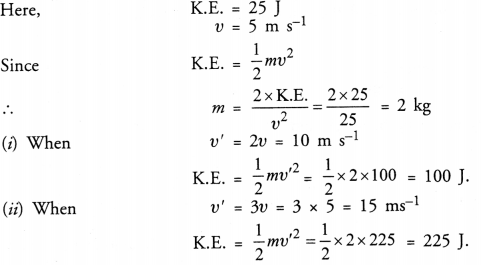Question 9.
What is power ?
Power is defined as the rate of doing work.

Question 10.
Define 1 Watt of power.
Define SI unit of power.
Power of an agent is said to be 1 watt if it does 1 joule work in 1 second.

Question 11.
A lamp consumes 1000 J of electrical energy in 10 s. What is its power ?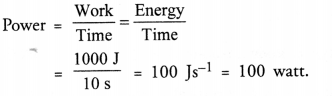Question 12.
Define average power.
Average power is defined as the ratio of total work done by an agent to the total time taken.

NCERT CHAPTER END EXERCISE

Question 1.
Look at the activities listed below. Reason out whether or not work is done in the light of your understanding of the term ‘work.’
(a) Suma is swimming in a pond.
(b) A donkey is carrying a load on its back.
(c) A wind mill is lilting water from a well.
(d) A great plant is carrying out photosynthesis.
(e) An engine is pulling a train.
(f) Food grains are getting dried in the sun.
(g) A sailboat is moving due to wind energy.
(a) Work is done (Reaction (Force) of water on Suma during swimming displaces Suma in the forward direction).
(b) No work is done (Force of gravity acting on the load is perpendicular to the displacement of the load).
(c) Work is done (Force displaces water in the upward direction).
(d) No work is done (There is no force and no displacement during photosynthesis).
(e) Work is done (Force displaces the train.)
(f) No work is done (There is no external force and no displacement of the grains.)
(g) Work is done (Force acting on the sail boat displaces the boat).

Question 2.
An object thrown at a certain angle to the ground moves in a curved path and falls back to the ground.
The initial and the final points of the path of the object lie on the same horizontal line. What is the work done by the force of gravity on the object ? (CBSE 2011, 2012)
Work done by the force of gravity on the object = mgh
Here, h = difference in height of the initial and final positions of the object.
Since, initial and final positions of the object are at the same level, so h = 0.
∴ Work done = mg x 0 = 0.

Question 3.
A battery lights a bulb. Describe the energy change involved in the process. (CBSE 2012)
The chemical energy of the battery changes into electrical energy which is then converted into light energy and heat energy.

Question 4.
Certain force acting on a 20 kg mass changes its velocity from 5 m s-1 to 2 m s-1. Calculate the work done by the force. (CBSE 2011, 2012)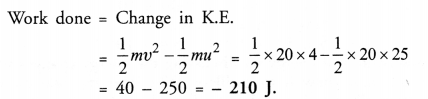Question 5.
A mass of 10 kg is at a point A on a table. It is moved to a point B which is at a distance of 2 m from A.
If the line joining A and B is horizontal, what is the work done on the object by the gravitational force ? Explain your answer. (CBSE 2012)
Work done by the gravitational force = mgh
Since h = 0 (because both points A and B are at the same height.)
Work done = 0.

Question 6.
The potential energy of a freely falling object decreases progressively. Does this violate the law of conservation of energy ? Why ? (CBSE 2011)
No, As the potential energy of a freely falling object decreases, the object acquires more and more speed. So the kinetic energy of the object increases at the cost of its potential energy. However, total energy of the object remains the same at every point.

Question 7.
What are the various energy transformations that occur when you are riding a bicycle ?
Our muscular energy is converted into the kinetic energy of the bicycle.

Question 8.
Does the transfer of energy takes place when you push a huge rock with all your might and fail to move it ? Where is the energy you spend going ?
When we push a huge rock, the rock also exerts a huge force on us. The muscular energy spent by us is used to oppose the huge force acting on us due to the rock.

Question 9.
A certain household has consumed 250 units of energy during a month. How much energy is this in joules ?Question 10.
An object of mass 40 kg is raised to a height of 5 m above the ground. What is its potential energy ? If the object is allowed to fall, find its kinetic energy when it is half-way down. (CBSE 2011)

1. Potential energy = mgh
= 40 kg x 10 m s-2 x 5 m = 2000 J.
2. According to the law of conservation of energy :
K.E. when it is half-way down = P.E. when it is half-way down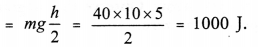Question 11.
What is the work done by the force of gravity on a satellite moving round the earth ? Justify your answer. (CBSE 2012)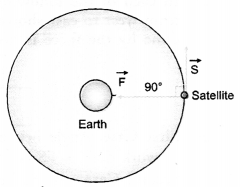The force of gravity acting on a satellite moves the satellite in a circular path. Since, the force of gravity acts at right angle to the displacement of the satellite (Figure), so work done,
W = FS cos 90° = 0

Question 12.
Can there be displacement of an object in the absence of any force acting on it ?
Yes, When an object moves with a constant velocity, then no force acts on the object. However, the object is displaced from one position to another position.

Question 13.
A person holds a bundle of hay over his head for 30 minutes and gets tired. Has he done some work or not? Justify your answer. (CBSE 2011, 2012)
Work = Force x Displacement.
Since the person is at rest, so displacement = 0.
Hence no work is done by the person.

Question 14.
An electric heater is rated 1500 W. How much energy does it use in 10 hours ? (CBSE 2011, 2012)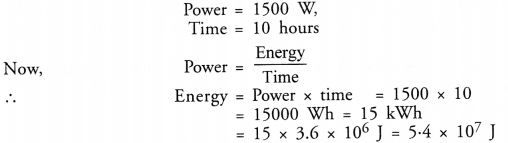Question 15.
Illustrate the law of conservation of energy by discussing the energy changes which occur when we draw a pendulum bob to one side and allow it to oscillate. Why does the bob eventually come to rest ? What happens to its energy eventually ? Is it a violation of the law of conservation of energy ?
For part

1. Consider a simple pendulum suspended from a rigid support at S. Let OS be the undisturbed position of the pendulum. Now, let the pendulum be displaced to position A where it is at rest (Figure 9).At position A, the pendulum has potential energy (mgh). When the pendulum is released from position A, it begins to move towards position O. The speed of the bob of the pendulum increases and its height decreases. So potential energy of the pendulum is changed into its kinetic energy. At position O, whole of the potential energy of the pendulum is converted to its kinetic energy.
2. When the bob of a simple pendulum oscillates in air, the air friction opposes its motion. The kinetic energy of the oscillating bob is used to overcome the air friction which tries to oppose the motion of the bob of the pendulum. As the oscillating bob strikes the air molecules, the kinetic energy of the bob of the pendulum is converted into heat energy. This heat energy is transferred to the atmosphere. In other words, the kinetic energy of the bob of the pendulum is converted into heat energy with the passage of time. Ultimately, the entire kinetic energy of the bob is converted into heat energy and hence the oscillating bob comes to rest. In this case, the law of conservation of energy is not violated as one form of energy is converted into another form of energy.

Question 16.
An object of mass m is moving with a constant velocity v. How much work should be done on the object in order to bring the object to rest ? (CBSE 2012)Question 17.
Calculate the work required to be done to stop a car of 1500 kg moving at a velocity of 60 km/h. (CBSE 2011, 2016)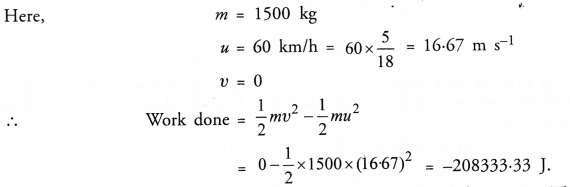Question 18.
In each of the following, a force F is acting on an object of mass, m. The direction of displacement is from west to east shown by the longer arrow. Observe the diagrams carefully and state whether the work done by the force is negative, positive or zero. (CBSE 2011)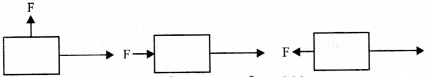First Case: Angle between force and displacement, 0 = 90°
∴ work done = FS cos 6 = FS cos 90° = 0
Second Case: Angle between force and displacement, 0 = 0°
work done = FS cos 9 = FS cos 0° = FS ( positive work) (∴ cos 0° = 1)
Third Case: Angie between force and displacement, 9 = 180°
work done = FS cos 9 = FS cos 180° = -FS ( negative work) (∴ cos 180° = -1)

Question 19.
Soni says that the acceleration in an object could be zero even when several forces are acting on it. Do you agree with him ? Why ?
Yes, If the sum of several forces acting on an object is zero, then net force acting on it is zero. Hence,Question 20.
Find the energy in kWh consumed in 10 hours by four devices of power 500 W each.
Total power, P = 500 W x 4 = 2000 W
Time, t = 10 h
Energy consumed = Power x Time
= 2000 W x 10 h = 20,000 Wh = 20 kWh.

Question 21.
A freely falling object eventually stops on reaching the ground. What happens to its kinetic energy ?
(CBSE 2011)